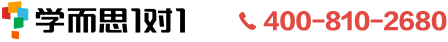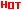<返回切换城市

## 北京小学数学公式大全

2016-04-18 15:11:26 来源：佚名## 免费领取新学期学习资料北京小学数学公式大全

小学数学一直是个难题，数学在孩子的整个学习生涯中的重要性不言而喻，今天爱智康小编就为同学们总结了小学数学公式大全，从小就打好数学基础，需要北京小学数学公式大全的同学看过来。

一、小学数学几何形体周长 面积 体积公式

长方形的周长=(长+宽)×2 C=(a+b)×2

正方形的周长=边长×4 C=4a

长方形的面积=长×宽 S=ab

正方形的面积=边长×边长 S=a.a= a

三角形的面积=底×高÷2 S=ah÷2

平行四边形的面积=底×高 S=ah

梯形的面积=(上底+下底)×高÷2 S=(a+b)h÷2

直径=半径×2 d=2r 半径=直径÷2 r= d÷2

圆的周长=圆周率×直径=圆周率×半径×2 c=πd =2πr

圆的面积=圆周率×半径×半径

三角形的面积=底×高÷2. 公式 S= a×h÷2

正方形的面积=边长×边长 公式 S= a×a

长方形的面积=长×宽 公式 S= a×b

平行四边形的面积=底×高 公式 S= a×h

梯形的面积=(上底+下底)×高÷2 公式 S=(a+b)h÷2

内角和：三角形的内角和=180度.

长方体的体积=长×宽×高 公式：V=abh

长方体(或正方体)的体积=底面积×高 公式：V=abh

正方体的体积=棱长×棱长×棱长 公式：V=aaa

圆的周长=直径×π 公式：L=πd=2πr

圆的面积=半径×半径×π 公式：S=πr2

圆柱的表(侧)面积：圆柱的表(侧)面积等于底面的周长乘高.公式：S=ch=πdh=2πrh

圆柱的表面积：圆柱的表面积等于底面的周长乘高再加上两头的圆的面积. 公式：S=ch+2s=ch+2πr2

圆柱的体积：圆柱的体积等于底面积乘高.公式：V=Sh

圆锥的体积=1/3底面×积高.公式：V=1/3Sh

分数的加、减法则：同分母的分数相加减,只把分子相加减,分母不变.异分母的分数相加减,先通分,然后再加减.

分数的乘法则：用分子的积做分子,用分母的积做分母.

分数的除法则：除以一个数等于乘以这个数的倒数.

二、单位换算

(1)1公里=1千米 1千米=1000米 1米=10分米 1分米=10厘米 1厘米=10毫米

(2)1平方米=100平方分米 1平方分米=100平方厘米 1平方厘米=100平方毫米

(3)1立方米=1000立方分米 1立方分米=1000立方厘米 1立方厘米=1000立方毫米

(4)1吨=1000千克 1千克= 1000克= 1公斤 = 2市斤

(5)1公顷=10000平方米 1亩=666.666平方米

(6)1升=1立方分米=1000毫升 1毫升=1立方厘米

(7)1元=10角1角=10分1元=100分

(8)1世纪=100年 1年=12月 大月(31天)有:1\3\5\7\8\10\12月 小月(30天)的有:4\6\9\11月

平年2月28天, 闰年2月29天 平年全年365天, 闰年全年366天 1日=24小时 1时=60分

1分=60秒 1时=3600秒

三、数量关系公式方面

1、每份数×份数=总数 总数÷每份数=份数总数÷份数=每份数

2、1倍数×倍数=几倍数 几倍数÷1倍数=倍数几倍数÷倍数=1倍数

3、速度×时间=路程 路程÷速度=时间 路程÷时间=速度

4、单价×数量=总价 总价÷单价=数量 总价÷数量=单价

5、工作效率×工作时间=工作总量 工作总量÷工作效率=工作时间工作总量÷工作时间=工作效率

6、加数+加数=和 和-一个加数=另一个加数

7、被减数-减数=差 被减数-差=减数 差+减数=被减数

8、因数×因数=积 积÷一个因数=另一个因数

9、被除数÷除数=商 被除数÷商=除数 商×除数=被除数

四、算术方面

1.加法交换律：两数相加交换加数的位置,和不变.

2.加法结合律：三个数相加,先把前两个数相加,或先把后两个数相加,再同第三个数相加,和不变.

3.乘法交换律：两数相乘,交换因数的位置,积不变.

4.乘法结合律：三个数相乘,先把前两个数相乘,或先把后两个数相乘,再和第三个数相乘,它们的积不变.

5.乘法分配律：两个数的和同一个数相乘,可以把两个加数分别同这个数相乘,再把两个积相加,结果不变.如：(2+4)×5=2×5+4×5.

6.除法的性质：在除法里,被除数和除数同时扩大(或缩小)相同的倍数,商不变.0除以任何不是0的数都得0.

7.等式：等号左边的数值与等号右边的数值相等的式子叫做等式.等式的基本性质：等式两边同时乘以(或除以)一个相同的数,等式仍然成立.

8.方程式：含有未知数的等式叫方程式.

9.一元一次方程式：含有一个未知数,并且未知数的次 数是一次的等式叫做一元一次方程式.

学会一元一次方程式的例法及.即例出代有χ的算式并.

10.分数：把单位“1”平均分成若干份,表示这样的一份或几分的数,叫做分数.

11.分数的加减法则：同分母的分数相加减,只把分子相加减,分母不变.异分母的分数相加减,先通分,然后再加减.

12.分数大小的比较：同分母的分数相比较,分子大的大,分子小的小.异分母的分数相比较,先通分然后再比较;若分子相同,分母大的反而小.

13.分数乘整数,用分数的分子和整数相乘的积作分子,分母不变.

14.分数乘分数,用分子相乘的积作分子,分母相乘的积作为分母.

15.分数除以整数(0除外),等于分数乘以这个整数的倒数.

16.真分数：分子比分母小的分数叫做真分数.

17.假分数：分子比分母大或者分子和分母相等的分数叫做假分数.假分数大于或等于1.

18.带分数：把假分数写成整数和真分数的形式,叫做带分数.

19.分数的基本性质：分数的分子和分母同时乘以或除以同一个数(0除外),分数的大小不变.

20.一个数除以分数,等于这个数乘以分数的倒数.

21.甲数除以乙数(0除外),等于甲数乘以乙数的倒数.

五、特殊问题

和差问题的公式

(和+差)÷2=大数

(和-差)÷2=小数

和倍问题

和÷(倍数-1)=小数

小数×倍数=大数

(或者 和-小数=大数)

差倍问题

差÷(倍数-1)=小数

小数×倍数=大数

(或 小数+差=大数)

今天爱智康小编的北京小学数学公式大全总结就是这些了，如果在学习数学方面有什么难题，可以拨打免费电话4000-121-121，会有专业老师为您解答哦!##体验预约丨学而思1对1/小班课程相关文章推荐